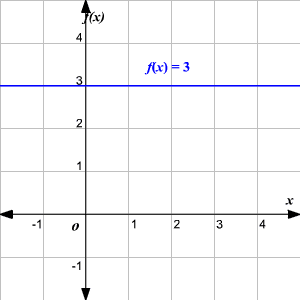# Constant Function

A constant function is a linear function for which the range does not change no matter which member of the domain is used.  $f\left({x}_{1}\right)=f\left({x}_{2}\right)$  for any ${x}_{1}$ and ${x}_{2}$ in the domain.

With a constant function, for any two points in the interval, a change in $x$ results in a zero change in $f\left(x\right)$ .

Example:

Graph the function $f\left(x\right)=3$ .The graph of a constant function is always a horizontal line .